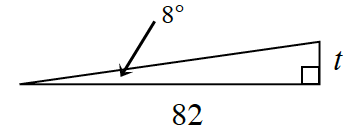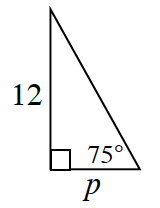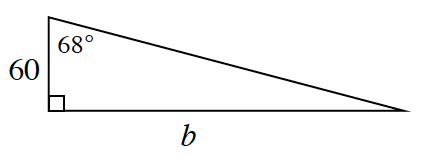### Home > CCG > Chapter 4 > Lesson 4.1.4 > Problem4-39

4-39.

Find the missing side length for each triangle. Use the tangent button on your calculator to help.

See the Math Notes box in Lesson 4.1.4 for additional help.

1.$\text{tan}(8º) =\frac{\Delta y}{\Delta x} = \frac{\textit{t}}{82}$

$\frac{\text{tan}(8º)}{1} = \frac{t}{82}$

$82(\tan8º)=t$

$t\approx11.524$

1.$p\approx3.215$

1.Rotate the triangle so that $68º$ is the slope angle, then use the same method as in parts (a) and (b).